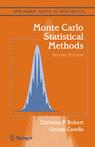## Typo in MCSM [bis]Doug Rivers from Stanford sent me the following email:

On p. 175 of Monte Carlo Statistical Methods, shouldn’t the last displayed equation just be$L(\theta|y) = \int_\mathcal{Z} L^c(\theta|y,z) \text{d}z$

I don’t see how you get$L(\theta|y) = \mathbb{E}[L^c(\theta|y,Z)]$.

Doug is completely right: the expectation, as written is incorrect. The difficulty with Example 5.14 was also pointed out in an earlier post. Alas, the resolution in this first post was  just as confusing as the mistake itself! (I have just updated the post to remove the confusion.) There is no expectation involved in this likelihood because the$z_i$‘s are truncated in a. Their density is therefore a renormalised version of$f(z-\theta)$… I now think the whole example should be rewritten because it starts as if m observations were uncensored out of n, only to move to a fixed censoring bound a. While both likelihoods are proportional when$a=y_m$, this confusion still is a bad idea!!!

This site uses Akismet to reduce spam. Learn how your comment data is processed.